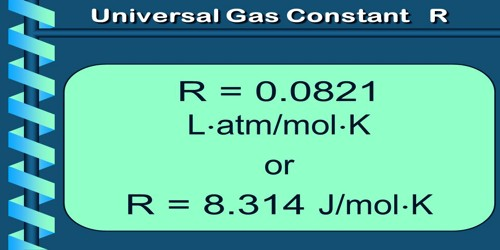# Universal Gas Constant

Universal gas constant

If the mass of any gas is one gram mole, then the value of K becomes same for all the gases and the constant K is expressed by R; that is why ‘R’ is called universal gas constant.

(a) Meaning of R: The value of R same for all gases. For this reason R is called universal gas constant. We know from ideal gas equation, PV = nRT.

or, R = PV/nT = [(work or energy) / (no of moles x temperature)] … … (1)

From equation (1), we can define R in the following way:

Definition: The work done by 1 mole of ideal gas if its temperature increases by l0 is called the universal gas constant.

(b) Unit of R: In S.I. method,

• unit of work or energy = Joule,
• unit of n = number of moles,
• unit of temperature = K (Kelvin).

So from equation (1), the unit of R is JK-1Mol-1.

(c) Value of R: In S.I. unit, at N.T.P.

P = 1.013 x 105 Nm-2.

The volume of 1 mole of a gas, V = 22.4 x 10-3 m-3 and T = 273.16 K

So, R = PV / nT = [(1.013 x 105) x (22.4 x 10-3)] / (1 x 273.16)

= 8.314 JK-1 mole-1.